# 七、对图像进行卡通化

• 如何访问网络摄像头
• 如何在实时视频流中进行键盘和鼠标输入
• 如何创建一个交互式应用
• 如何使用高级图像过滤器
• 如何将图像卡通化

# 访问网络摄像头

import cv2

cap = cv2.VideoCapture(0)

# Check if the webcam is opened correctly
if not cap.isOpened():
raise IOError("Cannot open webcam")

while True:
frame = cv2.resize(frame, None, fx=0.5, fy=0.5, interpolation=cv2.INTER_AREA)
cv2.imshow('Input', frame)

c = cv2.waitKey(1)
if c == 27:
break

cap.release()
cv2.destroyAllWindows()


## 底层原理

ret, frame = cap.read()


if c == 27:


# 键盘输入

import argparse

import cv2

def argument_parser():
parser = argparse.ArgumentParser(description="Change color space of the \
input video stream using keyboard controls. The control keys are: \
Grayscale - 'g', YUV - 'y', HSV - 'h'")
return parser

if __name__=='__main__':
args = argument_parser().parse_args()

cap = cv2.VideoCapture(0)

# Check if the webcam is opened correctly
if not cap.isOpened():
raise IOError("Cannot open webcam")

cur_char = -1
prev_char = -1

while True:
# Read the current frame from webcam

# Resize the captured image
frame = cv2.resize(frame, None, fx=0.5, fy=0.5, interpolation=cv2.INTER_AREA)

c = cv2.waitKey(1)

if c == 27:
break

if c > -1 and c != prev_char:
cur_char = c
prev_char = c

if cur_char == ord('g'):
output = cv2.cvtColor(frame, cv2.COLOR_BGR2GRAY)
elif cur_char == ord('y'):
output = cv2.cvtColor(frame, cv2.COLOR_BGR2YUV)

elif cur_char == ord('h'):
output = cv2.cvtColor(frame, cv2.COLOR_BGR2HSV)

else:
output = frame

cv2.imshow('Webcam', output)

cap.release()
cv2.destroyAllWindows()


# 鼠标输入

import cv2
import numpy as np

def detect_quadrant(event, x, y, flags, param):
if event == cv2.EVENT_LBUTTONDOWN:
if x > width/2:
if y > height/2:
point_top_left = (int(width/2), int(height/2))
point_bottom_right = (width-1, height-1)
else:
point_top_left = (int(width/2), 0)
point_bottom_right = (width-1, int(height/2))

else:
if y > height/2:
point_top_left = (0, int(height/2))
point_bottom_right = (int(width/2), height-1)
else:
point_top_left = (0, 0)
point_bottom_right = (int(width/2), int(height/2))

cv2.rectangle(img, (0,0), (width-1,height-1), (255,255,255), -1)
cv2.rectangle(img, point_top_left, point_bottom_right, (0,100,0), -1)

if __name__=='__main__':
width, height = 640, 480
img = 255 * np.ones((height, width, 3), dtype=np.uint8)
cv2.namedWindow('Input window')

while True:
cv2.imshow('Input window', img)
c = cv2.waitKey(10)
if c == 27:
break

cv2.destroyAllWindows()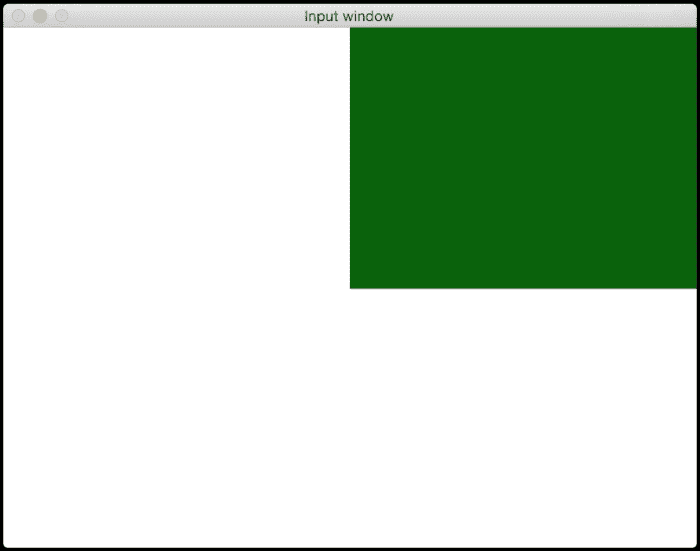## 下面发生了什么？

>>> import cv2
>>> print [x for x in dir(cv2) if x.startswith('EVENT')]


# 与实时视频流互动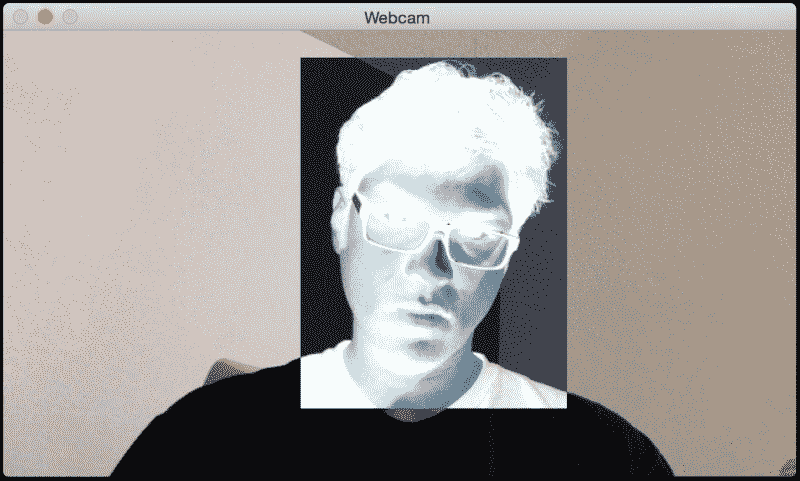import cv2
import numpy as np

def draw_rectangle(event, x, y, flags, params):
global x_init, y_init, drawing, top_left_pt, bottom_right_pt

if event == cv2.EVENT_LBUTTONDOWN:
drawing = True
x_init, y_init = x, y

elif event == cv2.EVENT_MOUSEMOVE:
if drawing:
top_left_pt = (min(x_init, x), min(y_init, y))
bottom_right_pt = (max(x_init, x), max(y_init, y))
img[y_init:y, x_init:x] = 255 - img[y_init:y, x_init:x]

elif event == cv2.EVENT_LBUTTONUP:
drawing = False
top_left_pt = (min(x_init, x), min(y_init, y))
bottom_right_pt = (max(x_init, x), max(y_init, y))
img[y_init:y, x_init:x] = 255 - img[y_init:y, x_init:x]

if __name__=='__main__':
drawing = False
top_left_pt, bottom_right_pt = (-1,-1), (-1,-1)

cap = cv2.VideoCapture(0)

# Check if the webcam is opened correctly
if not cap.isOpened():
raise IOError("Cannot open webcam")

cv2.namedWindow('Webcam')
cv2.setMouseCallback('Webcam', draw_rectangle)

while True:
img = cv2.resize(frame, None, fx=0.5, fy=0.5, interpolation=cv2.INTER_AREA)
(x0,y0), (x1,y1) = top_left_pt, bottom_right_pt
img[y0:y1, x0:x1] = 255 - img[y0:y1, x0:x1]
cv2.imshow('Webcam', img)

c = cv2.waitKey(1)
if c == 27:
break

cap.release()
cv2.destroyAllWindows()


## 我们是怎么做到的？

cv2.setMouseCallback('Webcam', draw_rectangle)


# 卡通化图像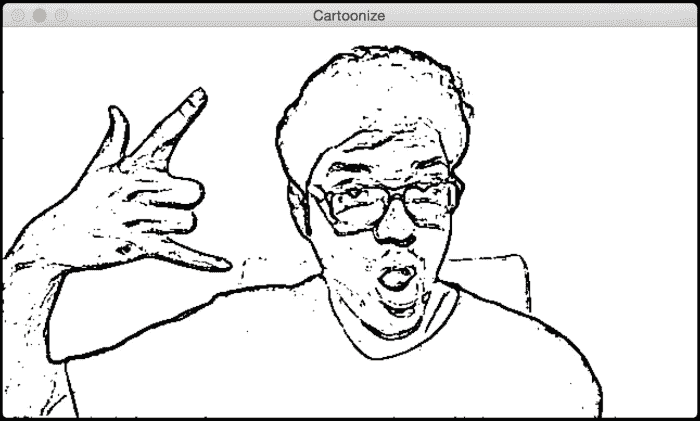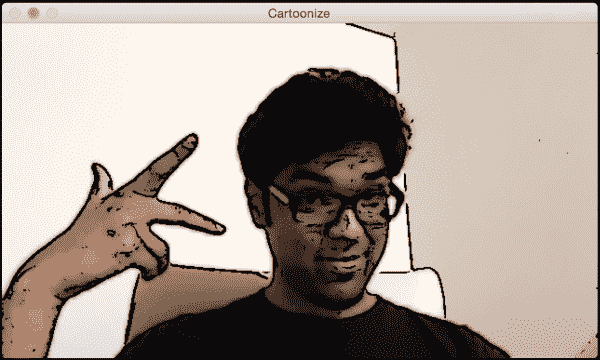import cv2
import numpy as np

def cartoonize_image(img, ds_factor=4, sketch_mode=False):
# Convert image to grayscale
img_gray = cv2.cvtColor(img, cv2.COLOR_BGR2GRAY)

# Apply median filter to the grayscale image
img_gray = cv2.medianBlur(img_gray, 7)

# Detect edges in the image and threshold it
edges = cv2.Laplacian(img_gray, cv2.CV_8U, ksize=5)
ret, mask = cv2.threshold(edges, 100, 255, cv2.THRESH_BINARY_INV)

# 'mask' is the sketch of the image
if sketch_mode:

# Resize the image to a smaller size for faster computation
img_small = cv2.resize(img, None, fx=1.0/ds_factor, fy=1.0/ds_factor, interpolation=cv2.INTER_AREA)
num_repetitions = 10
sigma_color = 5
sigma_space = 7
size = 5

# Apply bilateral filter the image multiple times
for i in range(num_repetitions):
img_small = cv2.bilateralFilter(img_small, size, sigma_color, sigma_space)

img_output = cv2.resize(img_small, None, fx=ds_factor, fy=ds_factor, interpolation=cv2.INTER_LINEAR)

dst = np.zeros(img_gray.shape)

# Add the thick boundary lines to the image using 'AND' operator
return dst

if __name__=='__main__':
cap = cv2.VideoCapture(0)

cur_char = -1
prev_char = -1

while True:
frame = cv2.resize(frame, None, fx=0.5, fy=0.5, interpolation=cv2.INTER_AREA)

c = cv2.waitKey(1)
if c == 27:
break

if c > -1 and c != prev_char:
cur_char = c
prev_char = c

if cur_char == ord('s'):
cv2.imshow('Cartoonize', cartoonize_image(frame, sketch_mode=True))
elif cur_char == ord('c'):
cv2.imshow('Cartoonize', cartoonize_image(frame, sketch_mode=False))
else:
cv2.imshow('Cartoonize', frame)

cap.release()
cv2.destroyAllWindows()


## 解构代码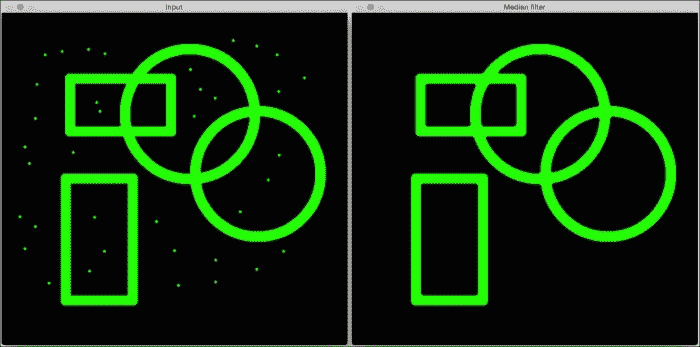import cv2
import numpy as np

output = cv2.medianBlur(img, 7)
cv2.imshow('Input', img)
cv2.imshow('Median filter', output)
cv2.waitKey()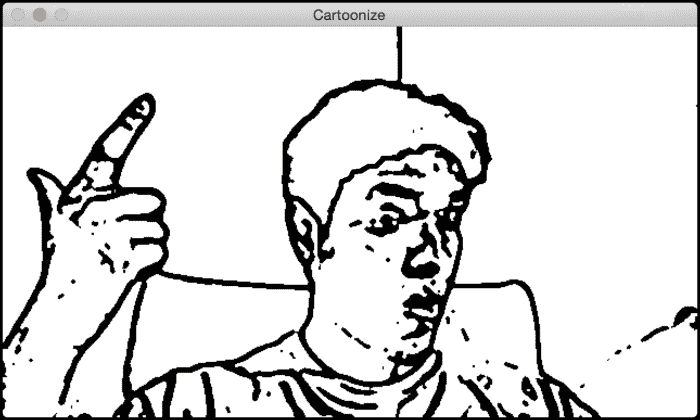if sketch_mode:
kernel = np.ones((3,3), np.uint8)
img_eroded = cv2.erode(img_sketch, kernel, iterations=1)
return cv2.medianBlur(img_eroded, 5)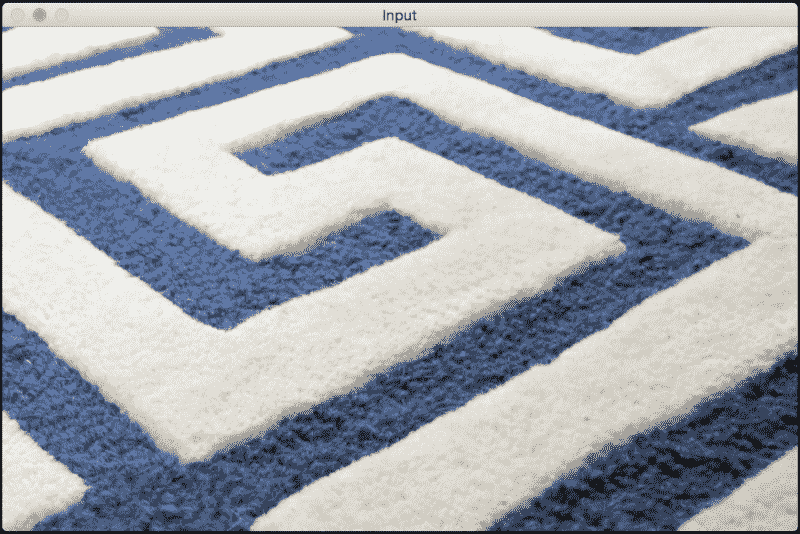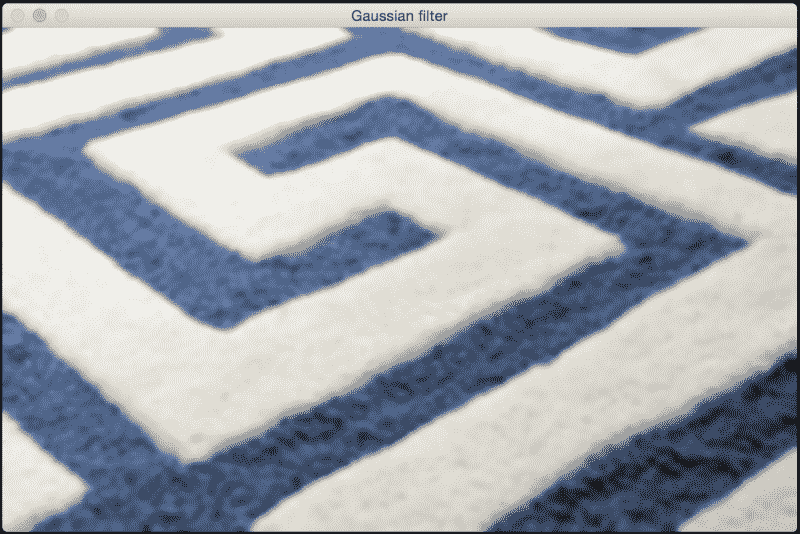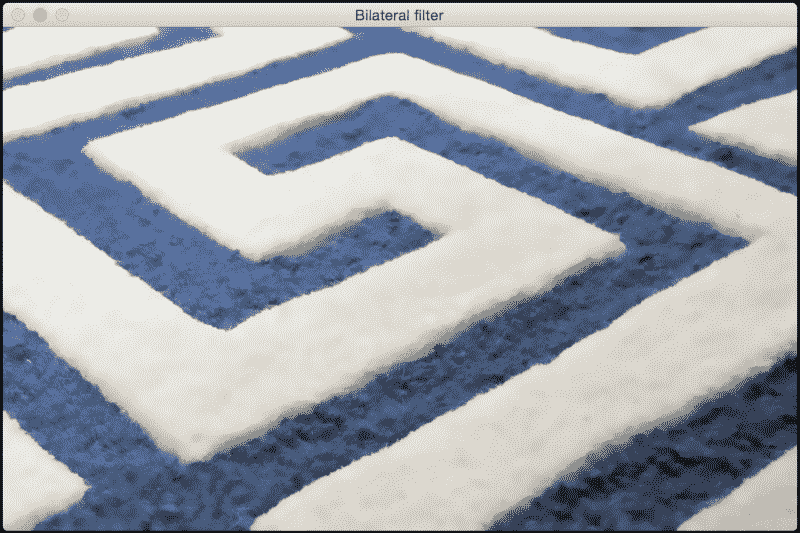import cv2
import numpy as np

img_gaussian = cv2.GaussianBlur(img, (13,13), 0)
img_bilateral = cv2.bilateralFilter(img, 13, 70, 50)

cv2.imshow('Input', img)
cv2.imshow('Gaussian filter', img_gaussian)
cv2.imshow('Bilateral filter', img_bilateral)
cv2.waitKey()


img_small = cv2.bilateralFilter(img_small, size, sigma_color, sigma_space)